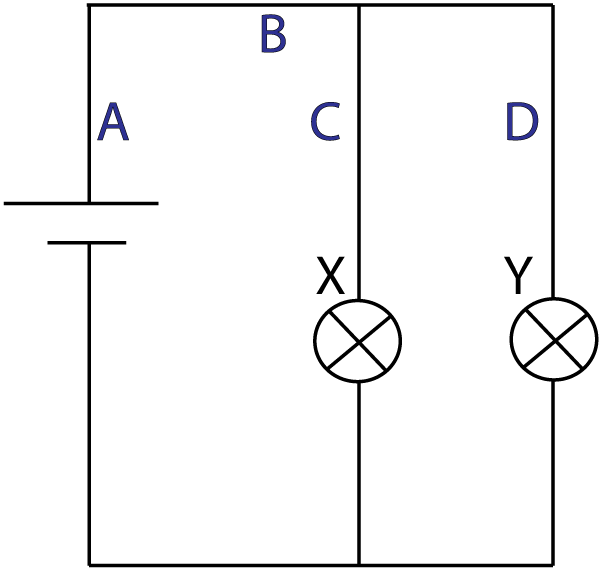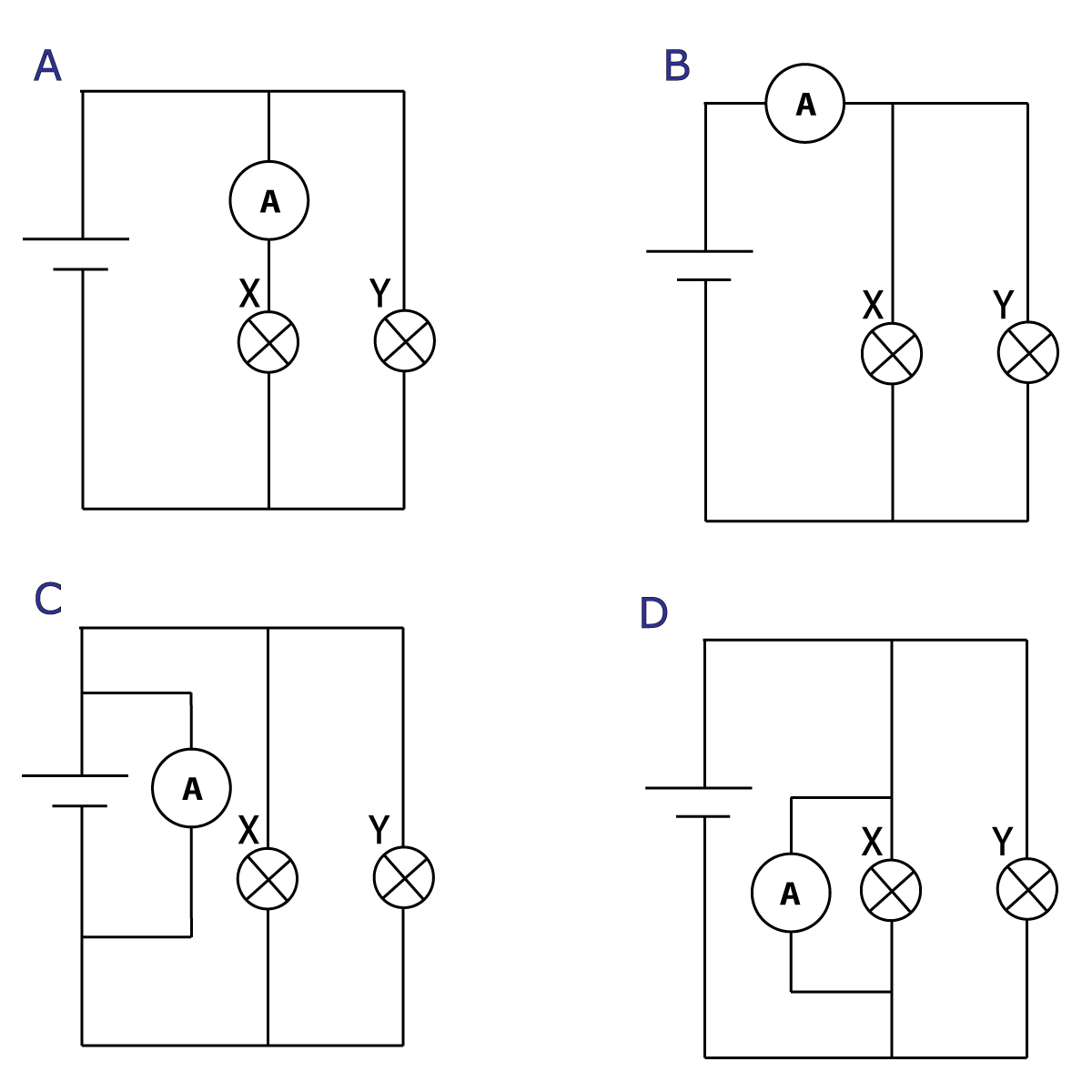10 minutes maximum! Can you do it in 5?

Q1-3. Look at these 4 circuit diagrams:Which of these ...

1. will not work?
2. is a series circuit?
3. is a parallel circuit?

4. A student wants to add a switch to the circuit below to turn bulb X on/off but leave bulb Y on. Where should it go?Q5+6: These questions are about current in circuits.

5. Which of these ammeters is in the right place to measure the current through bulb X?6. The current through bulb X shown in Q5 was measure to be 2 amps. What is the current through bulb Y, and what is the total current from the cell?

 Bulb Y Total current from cell A 2 A 2 A B 4 A 2 A C 2 A 4 A D 4 A 4 A
Q 7&8. Three identical bulbs, X, Y and Z are connected to a cell as shown.7. Which has the largest current through it?

• A. X
• B. Y
• C. Z
• D. all have the same current

8. In the circuit above the cell has a voltage of 12V. The potential difference (p.d.) across each bulb will be:

• A. X = 5V, Y = 4V, Z = 3V
• B. X = 4V, Y = 4V, Z = 4V
• C. X = 6V, Y = 6V, Z = 6V
• D. X = 12V, Y = 12V, Z = 12V

9. Which row in the table best describes how ammeters and voltmeters are connected in a circuit?

 Ammeter Voltmeter A series series B series parallel C parallel series D parallel parallel

10. Here is another circuit built using identical bulbs that all light up, but not all at the same brightness.Which of these bulbs will light up the brightest?